# Angles Around A Point Worksheet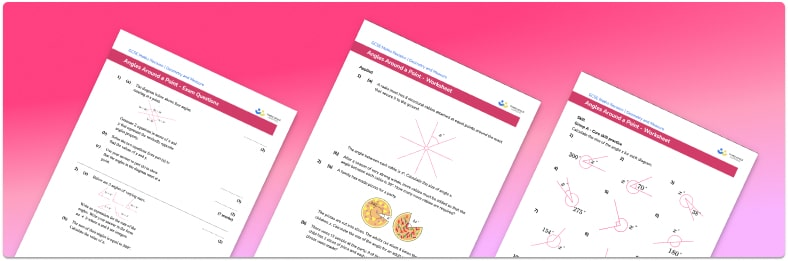• Section 1 of the angles around a point worksheet contains 36 skills-based angles around a point questions, in 3 groups to support differentiation
• Section 2 contains 4 applied angles around a point  questions with a mix of worded problems and deeper problem solving questions
• Section 3 contains 4 foundation and higher level GCSE exam style angles around a point questions
• Answer keys and a mark scheme for all angles around a point questions are provided
• Questions follow variation theory with plenty of opportunities for students to work independently at their own level
• All questions created by fully qualified expert secondary maths teachers
• Suitable for GCSE maths revision for AQA, OCR and Edexcel exam boards
• There are other printable angle properties worksheets with answer keys to accompany the angles around a point worksheets with plenty of practice finding missing angles, including angles in a quadrilateral and vertically opposite angles.

• This field is for validation purposes and should be left unchanged.

You can unsubscribe at any time (each email we send will contain an easy way to unsubscribe). To find out more about how we use your data, see our privacy policy.

### Angles around a point at a glance

An angle is formed when two lines or rays intersect at a vertex. Measuring angles with a protractor on a scale diagram is one way to find the size of unknown angles., however, a more efficient method is to use known angle facts when finding missing angles or calculating angles in 2D shape problems.

Angles around a point, or angles in a full turn, sum to 360°. So if, for example, we know that three angles ‘a’, ’b’ and ‘c’ lie around a point, and we know that angle ’a’ is a right angle, and we also know the size of angle ‘b’, we can find the size of angle ‘c’ by subtracting angle ‘b’ from 270°.

Looking forward, students can then progress to additional angles rules worksheets and other geometry worksheets, for example an angles in polygons worksheet or volume and surface area of spheres worksheet.For more teaching and learning support on Geometry our GCSE maths lessons provide step by step support for all GCSE maths concepts.

## Related worksheets

Vertically Opposite Angles Worksheet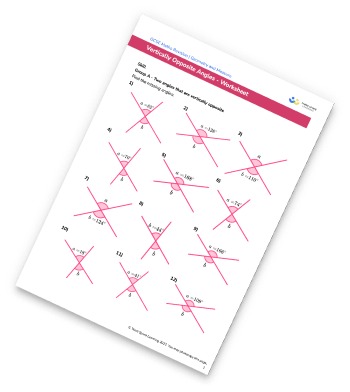Complementary And Supplementary Angles Worksheet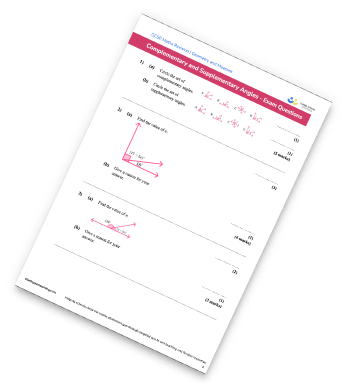Angles On A Straight Line Worksheet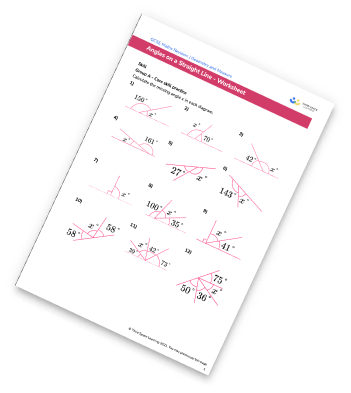Angle Rules Worksheet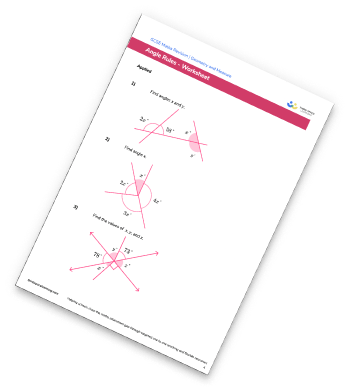## Do you have KS4 students who need more focused attention to succeed at GCSE?There will be students in your class who require individual attention to help them succeed in their maths GCSEs. In a class of 30, it’s not always easy to provide.

Help your students feel confident with exam-style questions and the strategies they’ll need to answer them correctly with our dedicated GCSE maths revision programme.

Lessons are selected to provide support where each student needs it most, and specially-trained GCSE maths tutors adapt the pitch and pace of each lesson. This ensures a personalised revision programme that raises grades and boosts confidence.

Find out more